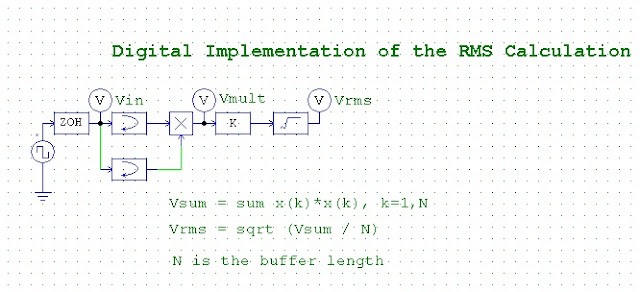# RMS voltage measurement with PSIM softwareHello friends, here is a very neat and nice simulation about RMS voltage measurement with PSIM simulation software.
The method described here is the simplest method to calculate RMS value of any signal.
Firstly the Zero order hold block is used to take the input from the source.
Then the source is multiplied by itself (producing square value)
Then the square root value is calculated by next block…..

if you change the source to a sine wave the output would be like this